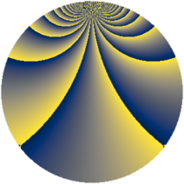# Properties

 Label 1728.1.bLevel $1728$ Weight $1$ Character orbit 1728.b Rep. character $\chi_{1728}(1567,\cdot)$ Character field $\Q$ Dimension $8$ Newform subspaces $2$ Sturm bound $288$ Trace bound $25$

# Related objects

## Defining parameters

 Level: $$N$$ $$=$$ $$1728 = 2^{6} \cdot 3^{3}$$ Weight: $$k$$ $$=$$ $$1$$ Character orbit: $$[\chi]$$ $$=$$ 1728.b (of order $$2$$ and degree $$1$$) Character conductor: $$\operatorname{cond}(\chi)$$ $$=$$ $$8$$ Character field: $$\Q$$ Newform subspaces: $$2$$ Sturm bound: $$288$$ Trace bound: $$25$$

## Dimensions

The following table gives the dimensions of various subspaces of $$M_{1}(1728, [\chi])$$.

Total New Old
Modular forms 48 8 40
Cusp forms 12 8 4
Eisenstein series 36 0 36

The following table gives the dimensions of subspaces with specified projective image type.

$$D_n$$ $$A_4$$ $$S_4$$ $$A_5$$
Dimension 8 0 0 0

## Trace form

 $$8q + O(q^{10})$$ $$8q - 4q^{25} + 8q^{73} - 8q^{97} + O(q^{100})$$

## Decomposition of $$S_{1}^{\mathrm{new}}(1728, [\chi])$$ into newform subspaces

Label Dim. $$A$$ Field Image CM RM Traces $q$-expansion
$$a_2$$ $$a_3$$ $$a_5$$ $$a_7$$
1728.1.b.a $$4$$ $$0.862$$ $$\Q(\zeta_{12})$$ $$D_{6}$$ $$\Q(\sqrt{-6})$$ None $$0$$ $$0$$ $$0$$ $$0$$ $$q+(-\zeta_{12}^{2}-\zeta_{12}^{4})q^{5}-\zeta_{12}^{3}q^{7}+\cdots$$
1728.1.b.b $$4$$ $$0.862$$ $$\Q(\zeta_{12})$$ $$D_{6}$$ $$\Q(\sqrt{-3})$$ None $$0$$ $$0$$ $$0$$ $$0$$ $$q-\zeta_{12}^{3}q^{7}+(-\zeta_{12}^{2}-\zeta_{12}^{4})q^{13}+\cdots$$

## Decomposition of $$S_{1}^{\mathrm{old}}(1728, [\chi])$$ into lower level spaces

$$S_{1}^{\mathrm{old}}(1728, [\chi]) \cong$$ $$S_{1}^{\mathrm{new}}(576, [\chi])$$$$^{\oplus 2}$$